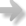﻿ Calculations > Equations > Duct Size Equations > Duct Surface Area Equations

# Duct Surface Area Equations

Navigation:  Calculations > Equations > Duct Size Equations >

# Duct Surface Area EquationsRound: Area = Pi * (D / 12) * L

Where:

Area        = Surface area of duct in square feet

Pi        = 3.14159265

D        = Diameter of duct in inches

12        = Inches per foot

L        = Length of duct in feet

Rectangular: Area = (W * 2 + H * 2) / 12 * L

Where:

Area        = Surface area of duct in square feet

W        = Width of duct in inches

H        = Height of duct in inches

12        = Inches per foot

L        = Length of duct in feet

Flat Oval: Area = (Pi * H + 2 * W) / 12 * L

Where:

Area        = Surface area of duct in square feet

Pi        = 3.14159265

W        = Width of duct in inches

H        = Height of duct in inches

12        = Inches per foot

L        = Length of duct in feet